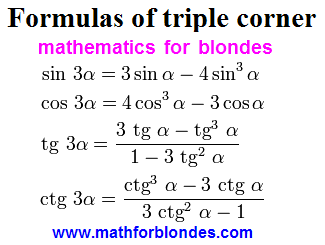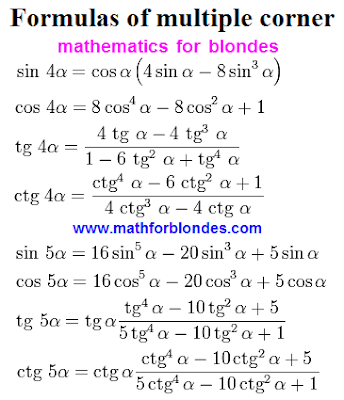## 9/12/2012

### Trigonometry formulas multiple anglesFormulas in trigonometry multiple angles enjoy. There is a sine, cosine, tangent and cotangent double, triple, multiple arguments. In simple words, sin, cos, tg, ctg two alpha, alpha three, four and five alpha.

For all of the double angle formulas are written continuously through equality. In simplifying trigonometric expressions, these formulas taken as read from left to right and right to left in any order. Such a method of making the right formula is called the "method of sewing." Cut a piece of it, there is a piece, sewn together by an equal sign = Get ready-made formula. Cut to full expression, from one of the equal sign to the other. The drafters of the tasks do so. Or something like that then can think of to confuse you more.

Why are these formulas of trigonometry? To simplify trigonometric expressions, which complicated the task compilers. Perhaps they need more in some cases, but it's hard to come up with such. It is necessary to ask the physicists and engineers, whether they had to use these formulas.